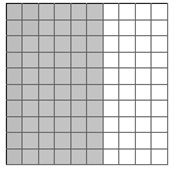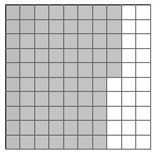# Representing Benchmark Percentages on a Grid

Benchmark percentages are rounded percentages that we often use. 1%, 10%, 25%, $\frac{331}{3}\%$ and their multiples are some benchmark percentages

We sometimes use a benchmark percentage that is close to a given percentage to estimate. Here are some common benchmark percentages and their fractional equivalents.

S.No Fraction Benchmark Percentage
1

$\frac{1}{10}$

10%
2

$\frac{1}{5}$

20%
3

$\frac{1}{4}$

25%
4

$\frac{1}{2}$

50%
5

$\frac{3}{4}$

75%
6 1 100%

To estimate 47%, you could use 50% as a benchmark percent.

Represent the benchmark percentage of the following percentage on a grid.

24%

### Solution

Step 1:

We consider a 10 × 10 grid having 100 squares.

The number of shaded squares gives the percentage of the grid as that is out of a total of 100 squares.

Step 2:

The benchmark percentage of 21% is 20%. To show 20% on the grid, we show 20 shaded squares as shown below.Represent the benchmark percentage of the following percentage on a grid.

59%

### Solution

Step 1:

We consider a 10 × 10 grid having 100 squares.

The number of shaded squares gives the percentage of the grid as that is out of a total of 100 squares.

Step 2:

The benchmark percentage of 59% is 60%. To show 60% on the grid, we show 60 shaded squares as shown below.Represent the benchmark percentage of the following percentage on a grid.

74%

### Solution

Step 1:

We consider a 10 × 10 grid having 100 squares.

The number of shaded squares gives the percentage of the grid as that is out of a total of 100 squares.

Step 2:

The benchmark percentage of 74% is 75%. To show 75% on the grid, we show 75 shaded squares as shown below.